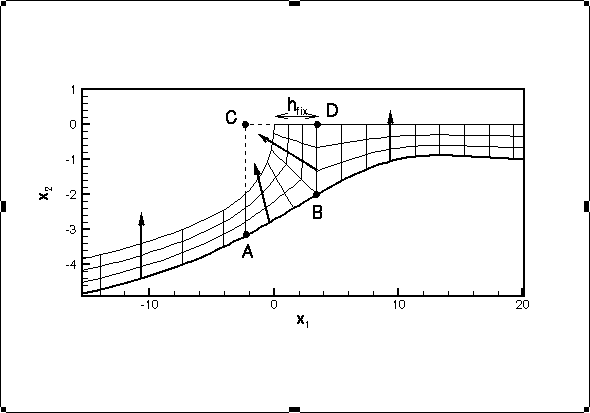Airway Reopening: The propagation of an air finger into a collapsed elastic tube

The computational model

The mathematical model of airway reopening comprises the free surface Navier-Stokes equations which describe the fluid flow, coupled to the equations of geometrically non-linear elasticity which describe the large deformations of the airway wall.

The equations were discretised by finite element methods: Displacement based finite elements were used to discretise the wall equations which were formulated in Lagrangian coordinates; the discretisation of the Navier-Stokes equations (formulated in spatially fixed, Eulerian coordinates) was achieved by utilising a moving mesh which adapted itself in response to the wall deformation. The method of spines was used for the automatic mesh update. The figure below shows the structure of the mesh (the symmetry of the configuration was exploited by only discretising one half of the domain) -- the actual computations were carried out with a much finer resolution.The discretised fluid and solid equations were solved in a fully coupled manner, using a Newton-Raphson method with adaptive parameter incrementation.

Back to `Airway Reopening'.